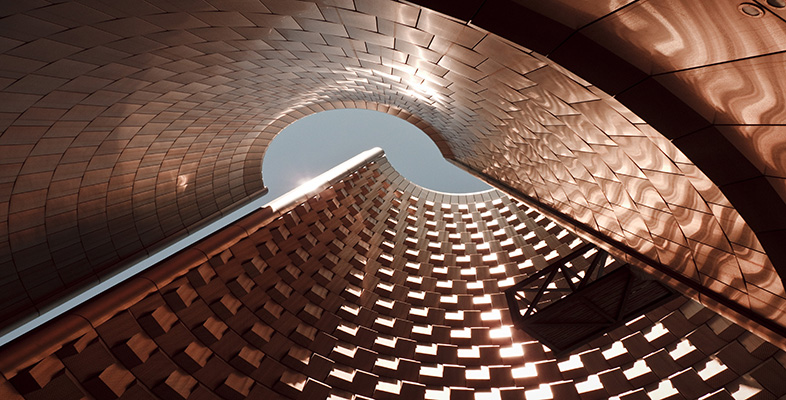Surfaces

Start this free course now. Just create an account and sign in. Enrol and complete the course for a free statement of participation or digital badge if available.

Free course

# 2.3.1 Cylinder

The simplest example of a paper-and-glue construction is to make a rectangle into a cylinder by gluing together two opposite edges. We take a closed rectangle ABB' A' in the plane and identify the opposite edges AB and A'B', as shown in Figure 27. This means that:

• we imagine A and A' to be the same point (which we'll label A);

• we imagine B and B' to be the same point (which we'll label B);

• we match up all corresponding pairs of points in between (such as P and P') as shown.

The direction of the identification is given by the order of the letters labelling an edge: labelling an edge AB implies a direction from A to B; similarly A'B' implies a direction from A' to B'.

Note that we have drawn arrowheads on the edges AB and A'B' of the rectangle to emphasise that they are to be identified in the same direction.

We also obtain a cylinder if we identify the edges AA' and BB'.

M338_1